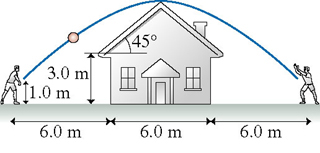# Problem: You're 6.0 m from one wall of a house. You want to toss a ball to your friend who is 6.0 m from the opposite wall. The throw and catch each occur 1.0 m above the ground. Assume the overhang of the roof is negligible, so that you may assume the edge of the roof is 6.0 m from you and 6.0 m from your friend.(a) What minimum speed will allow the ball to clear the roof?(b) At what angle should you toss the ball?

###### FREE Expert Solution
92% (224 ratings)
###### Problem Details

You're 6.0 m from one wall of a house. You want to toss a ball to your friend who is 6.0 m from the opposite wall. The throw and catch each occur 1.0 m above the ground. Assume the overhang of the roof is negligible, so that you may assume the edge of the roof is 6.0 m from you and 6.0 m from your friend.
(a) What minimum speed will allow the ball to clear the roof?
(b) At what angle should you toss the ball?